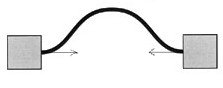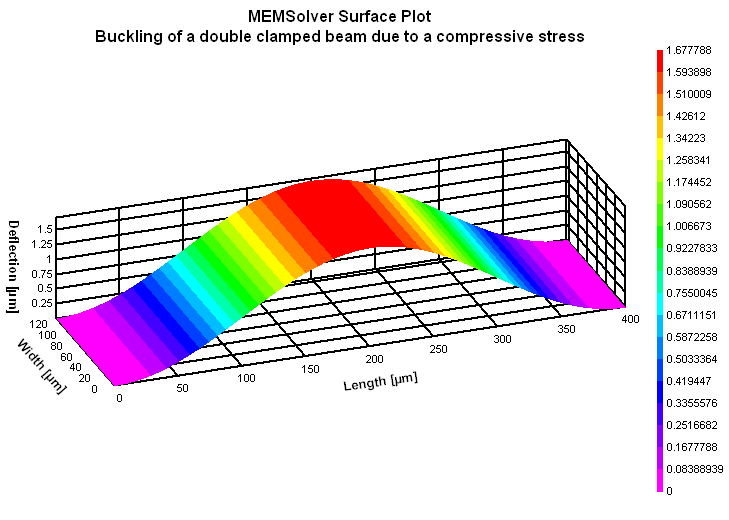BUCKLE,len,wid,thc,str,ymod,sel
Buckling of a double clamped beam

len          length of beam in µm
wid         width of beam in µm
thc          thickness of beam in µm
str          compressive stress acting on the beam in MPa
ymod      Young's modulus of the material in GPa
sel          number denoting the selected result.
Use 1 for buckling force, 2 for maximum deflection and 3 for buckling stressNotes

If a double clamped beam as shown in the figure above is subjected to a compressive stress, it will buckle if the applied stress is higher than the critical buckling stress of the beam. Beyond the critical buckling force, the beam will not be stable in the straight position and will assume a cosine shape. This is the first order buckling.

Use this form to determine the critical buckling force and corresponding buckling stress for the given beam. If the compressive stress is known, the deflection resulting from buckling can be estimated. This is the maximum deflection value at the center of the beam.

The plot shows the deflection profile for the given compressive stress. Using the crosshair tool, the deflection at every point on the beam can be estimated.Assumptions

-The default material is Silicon with a Young's modulus of 180GPa.
-The beam has uniform cross section.
-The fixed ends are perfectly clamped.
-A cosine shape for the buckled beam is assumed.
-The compressive force acts along the middle plane of the beam.